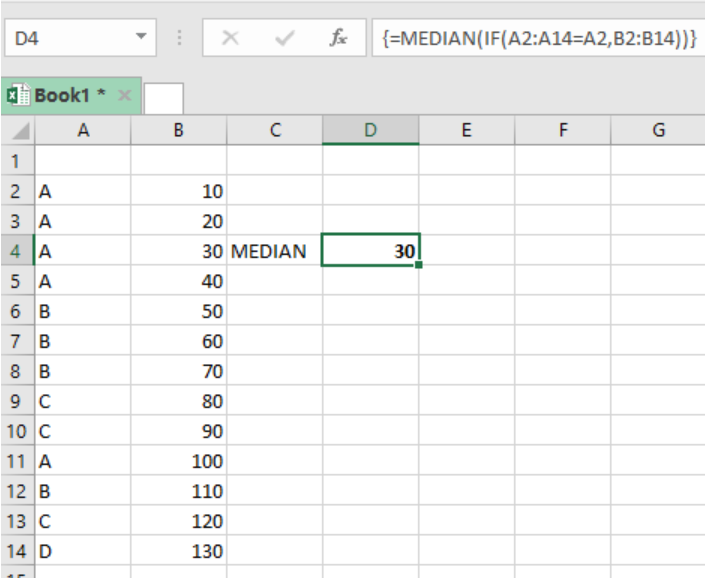Get instant live expert help with Excel or Google Sheets“My Excelchat expert helped me in less than 20 minutes, saving me what would have been 5 hours of work!”

#### Post your problem and you’ll get expert help in seconds.

Your message must be at least 40 characters
Our professional experts are available now. Your privacy is guaranteed.

# Learn How to Use MEDIAN and IF to Find the Median When There are Conditions

With knowledge of excel MEDIAN and IF functions, it is now possible to get the median of values that meet a given criteria. Note that excel does not have MEDIANIF as a function. But by combining these two functions, we can be able to achieve our goals. This post provides a clear guide on how to use a combination of MEDIAN and IF functions to get the median when there are conditions.Figure 1. Final result

## General syntax of the formula

`{=MEDIAN(IF(range=criteria, median_range))}`

## Understanding the formula

This formula is a combination of two functions, the MEDIAN and IF functions. Note that we do not have a single function in excel that combines MEDIAN and IF functions.

But for us to get the median based on set conditions, we need to use both of these functions.

In the example above, our criteria is to get median based on item named A. column A shall be our range, while B will be the median_range.

We then set our formula as shown below;

`{=MEDIAN(IF(A2:A14=A2,B2:B14))}`

It is important to remember that this is an array formula, thus needs to be entered by Control + Shift + Enter.

## Instant Connection to an Expert through our Excelchat Service

Most of the time, the problem you will need to solve will be more complex than a simple application of a formula or function. If you want to save hours of research and frustration, try our live Excelchat service! Our Excel Experts are available 24/7 to answer any Excel question you may have. We guarantee a connection within 30 seconds and a customized solution within 20 minutes.

Solution exampleshello i need help creating a formula, if a value does not exsits put 0, if a value exist do nothing
Solved by M. F. in 9 minsCompute the weighted average of students score from a spreadsheet using different weight for each category of marks
Solved by G. E. in 20 minshi there, im making a spread sheet to track progress in a game and would like to create a drop log sheet, i was hoping to use the if function to detect weather a item is logged on a sheet then send the sheet number to a list if so
Solved by K. F. in 60 minsI have a schedule I'm doing with formulas to add workdays (across rows) to a start date column. I would like to write the equation like this: =ifError((workday(b2,1) = cell above, [value of cell above + 1] Help me form this correctly to get the (value of target cell) = (1 more than the value from the cell above) IF the target cell value started equal to the cell above.
Solved by M. J. in 20 minsI need a formula that will subtract the sum of one cell if the sum of another cell is greater than zero, and have it applied to cells across a document that is 30,000 entries and only in certain spots
Solved by F. J. in 20 mins